Reference: T. Mitchell, 1997.

Inductive inference is the process of reaching a general conclusion from specific examples.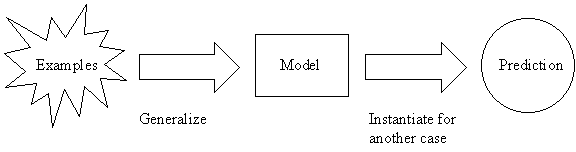The general conclusion should apply to unseen examples.

Inductive Learning Hypothesis: any hypothesis found to approximate the target function well over a sufficiently large set of training examples will also approximate the target function well over other unobserved examples.

Example:

Identified relevant attributes: x, y, z

 x y z 2 3 5 4 6 10 5 2 7

Model 1:

x + y = z

Prediction: x = 0, z = 0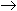y = 0

Model 2:

if x = 2 and z = 5, then y = 3.
if x = 4 and z = 10, then y = 6.
if x = 5 and z = 7, then y = 2.
otherwise y = 1.

Model 2 is likely overfitting.

 Good: completely consistent with data. Bad: no justification in the data for the prediction that y = 1 in all other cases. not in the class of algebraic functions (but nothing was said about class of descriptions).

Inductive bias: explicit or implicit assumption(s) about what kind of model is wanted.

Typical inductive bias:

• prefer models that can be written in a concise way.
• Select the shortest one.

Example:

• The inductive bias of the decision tree ID3 algorithm is a preference for certain hypotheses over others (e.g., for shorter hypotheses), with no hard restriction on the hypotheses that can be eventually enumerated. This form of bias is typically called a preference bias (or, alternatively, a search bias).
• In contrast, the bias of the version space candidate-elimination algorithm is in the form of a categorical restriction on the set of hypotheses considered. This form of bias is typically called a restriction bias (or, alternatively, a language bias).
• Typically, a preference bias is more desirable than a restriction bias because it allows the learner to work within a complete hypothesis space that is assured to contain the unknown target function.
• A restriction bias that strictly limits the set of potential hypotheses is generally less desirable because it introduces the possibility of excluding the unknown target function altogether.

Some languages of interest:

• Conjunctive normal form for Boolean variables A, B, C.
• e.g., ABC
• Disjunctive normal form.
• e.g., A(~B)(~C) + A(~B)C + AB(~C)
• Algebraic expressions.

Positive and Negative Examples

Positive Examples

• Are all true.

 x y z 2 3 5 2 5 7 4 6 10
 general x, y, z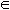I more specific x, y, zI+ more specific than the first two 1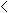x, y, z11 ; x, y, zI even more specific model x + y = z

Negative Examples

• Constrain the set of models consistent with the examples.

 x y z Decision 2 3 5 Y 2 5 7 Y 4 6 10 Y 2 2 5 N

Search for Description

Description keeps getting larger or longer.

Finite language - algorithm terminates.

Infinite language - algorithm runs

• Forever.
• Until out of memory.
• Until a final answer is reached.

X = example space/instance space (all possible examples)

D = description space (set of descriptions defined as a language L)

• Each description lL corresponds to a set of examples Xl.Success Criterion

Look for a description lL such that l is consistent with all observed examples.

• description can be relaxed to match all instances.
• inductive bias can be expressed in the success criterion.

Example:

L = {x op y = z}, op = {+, -, *, /}

Given a precise specification of language and data, write a program to test descriptions one by one against the examples.

• finite language: size = |L|
• finite number of examples: size = |X|
•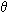(|L| * |X|)

Why is Machine Learning Hard (Slow)?

It is very difficult to specify a small finite language that contains a description of the examples.

e.g., algebraic expressions on 3 variables is an infinite language